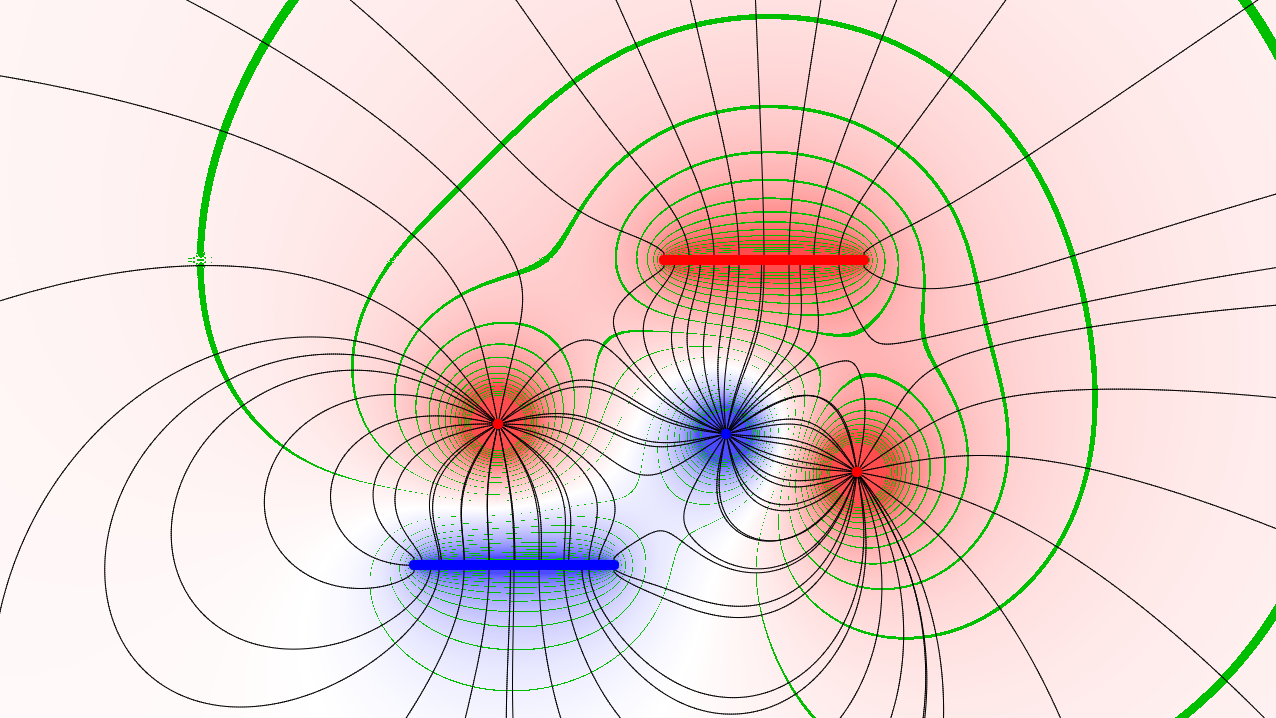# Visualising Electric Fields with WebGL December 14, 2017I recently saw a few blog posts (such as this), which inspired me to do something with WebGL. In Physics class we just finished learning about electric and magnetic fields, and I wanted to see what electric field lines would actually look like, as opposed to something that I just drew on paper. My visualisation can be seen here.

# Electric Field Lines

An electric field is a vector field, where each point in space has a direction and magnitude attached to it. In an electric field, these vectors represent the force that a charged object (1C) would experience when placed in that position. To illustrate this vector field, electric field lines are drawn emanating from a charge, which represents the path a charge would take, given an initial position, where the line starts.

My visualisation of this uses a normal 2D canvas for this (no WebGL yet!). A function called getStrength calculates the electric force at a given position. This calculation relies the superposition principle, where we sum up all the forces created by each charge in the simulation.

### Point Charges

Each point charge creates a force F: $F = \frac{kqq_0}{r^2}$, where $$q$$ is the charge of the source, $$q_0 = 1C$$, and $$r$$ is the distance from the charge to the position considered. Then initial starting points for paths are created, radially dividing point charges into divisions. These paths are then calculated using Euler's method.

### Plate Charges

A plate charge is more difficult to calculate, as it is composed of infinitely many point charges of charge 0, which add up to a charge $$q$$. To compute the force exerted by a plate, we split up the plate into $$n$$ point charges each with charge $$q \over n$$, and compute the force exerted by these charges as a whole.

$F = \sum^n_{i = 0} \frac{k\frac{q}{n}}{r_i^2}$

To extend this to 2D (forces are vectors, not scalars), we consider the vertical and horizontal components of this force separately. On each of those, we take the limit as $$n$$ approaches infinity, resulting in an integral.

The force parallel to the plate results in $F= \frac{kq}{l} \int_0^l \frac{r - p}{((r-p^2) + d^2)^\frac{3}{2}} dp$, where $$l$$ is the length of the plate, $$r$$ is the distance to the left endpoint of the plate (in direction parallel to the plate), and $$d$$ is the distance to the plate in the direction perpendicular to the plate. (If only I knew linear algebra and vector calculus better...)

The expansion and vertical component of the force is left as an exercise to the reader.

# Euler's method

Given an initial position $$s_0$$, we calculate the force $$F_0$$ at that position using getStrength. By Newton's 2nd law we have $F_0 = \frac{d^2s}{dt^2}$ for a unit mass. After a small step $$dt$$, the force will have moved this unit mass to a position $s_1 = F_0 * dt$. From here we calculate $$F_1$$, again with getStrength, where we calculate a new position $$s_2$$. This process is repeated until a limit (currently 1000 in my program), where each position $$s$$ is a point on the path.

# Electric Potential

Finally we get to the WebGL part! Since electric potential is a scalar value, I thought it would be a cool idea to show the electric potential around these charges using colours. To do this, we use a fragment shader where each fragment is coloured by the electric potential at that point. Electric potential is similar to electric field strength, but it represents the potential energy for a unit charge at a position and thus is directly inversely proportional to the distance.

$V = \frac{kqq_0}{r}$

The calculations are all very similar to before, but this time there are no vector components!

For a plate charge the electric potential is calculated similarly to the electric field. There is only one scalar component now:

$V = \frac{kq}{l} \int_0^l \frac{1}{\sqrt{d^2 + (r- p)^2}} dp$

From here, we can evaluate and simplify the definite integral, giving us: $V = \ln\left(\frac{\sqrt{(l-r)^2 + d^2} + r - l}{\sqrt{r^2 + d^2} + r}\right)$.

Using this formula for a plate charge and the previous formula for a point charge, we calculate the total electric potential $$\sum V$$ at a point. This point is then assigned a colour based on sign and magnitude, and rendered to the screen, all in a fragment shader!

Credits to Jacky Liao who helped with a bunch of math and stuff!

This is my first blog post.